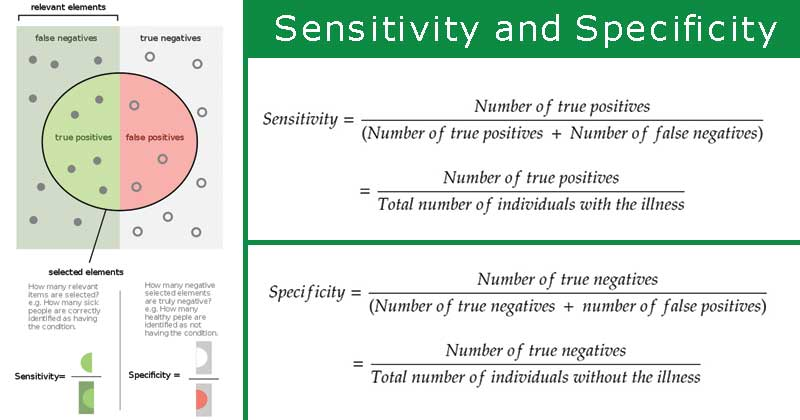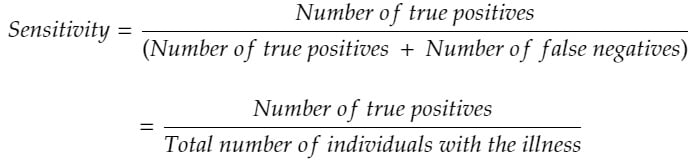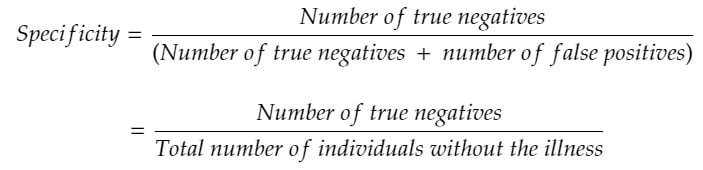# Sensitivity and Specificity

Watch Biology Educational Videos

Last Updated on January 3, 2020 by Sagar Aryal

# Sensitivity and Specificity

• When developing diagnostic tests or evaluating results, it is important to understand how reliable those tests and therefore the results obtained are.
• By using samples of known disease status, values such as sensitivity and specificity can be calculated that allow its evaluation.
• Therefore, when evaluating diagnostic tests, it is important to calculate the sensitivity and specificity for that test to determine its effectiveness.Image Source: Wikipedia

## Sensitivity

• The term sensitivity was introduced by Yerushalmy in the 1940s as a statistical index of diagnostic accuracy.
• It is also called the true positive rate, the recall, or probability of detection.
• It has been defined as the ability of a test to identify correctly all those who have the disease, which is “true-positive”.
• A 90 percent sensitivity means that 90 percent of the diseased people screened by the test will give a “true-positive” result and the remaining 10 percent a “false-negative” result.
• Thus, a highly sensitive test rarely overlooks an actual positive (for example, showing “nothing bad” despite something bad existing).

## Calculating Sensitivity

• The sensitivity of a diagnostic test is expressed as the probability (as a percentage) that a sample tests positive given that the patient has the disease.
• The following equation is used to calculate a test’s sensitivity:

##Specificity

• It is defined as the ability of a test to identify correctly those who do not have the disease, that is, “true-negatives”.
• It is also called as the true negative rate.
• A 90 percent specificity means that 90 percent of the non-diseased persons will give a “true-negative” result, 10 percent of non-diseased people screened by the test will be wrongly classified as “diseased” when they are not.
• Thus, a highly specific test rarely registers a positive classification for anything that is not the target of testing.

## Calculating Specificity

The specificity of a test is expressed as the probability (as a percentage) that a test returns a negative result given that that patient does not have the disease.

The following equation is used to calculate a test’s specificity:

##Relationship between Sensitivity and Specificity

• In medical tests, sensitivity is the extent to which actual positives are not overlooked (so false negatives are few), and specificity is the extent to which actual negatives are classified as such (so false positives are few).
• Although a screening test ideally is both highly sensitive and highly specific, we need to strike a balance between these characteristics, because most tests cannot do both.
• We determine this balance by an arbitrary cut-off point between normal and abnormal.
• If we want to increase sensitivity and to include all true positives, we are obliged to increase the number of false positives, which means decreasing specificity.
• Reducing the strictness of the criteria for a positive test can increase sensitivity, but by doing this the test’s specificity is reduced.
• Likewise, increasing the strictness of the criteria increases specificity but decreases sensitivity.

This site uses Akismet to reduce spam. Learn how your comment data is processed.